## QCD

### Why Colour?

There are several strong evidences why quarks should carry - besides the electric charge - an additional internal degree of freedom: the colour charge.
1. Quark Wave Function of Baryons
2. Electron Positron Annihilation into Hadrons
3. tau decays
Tau leptons may decay weakly into pure leptonic final states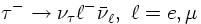or into hadronic final states plus a tau neutrino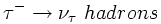At tree level the Feynman diagrams for these decays look likeNeglecting particle masses in the final state and assuming lepton universality (i.e. the weak coupling constant is the same for leptons and quarks) the rates for all these processes are equal.

As a consequence, the leptonic branching fractions are predicted to be: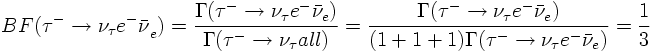If one assumes that quarks cary Nc colour charges the prediction is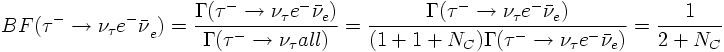The measured values are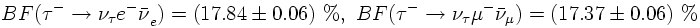which strongly supports Nc=3.
4. pi0 decay